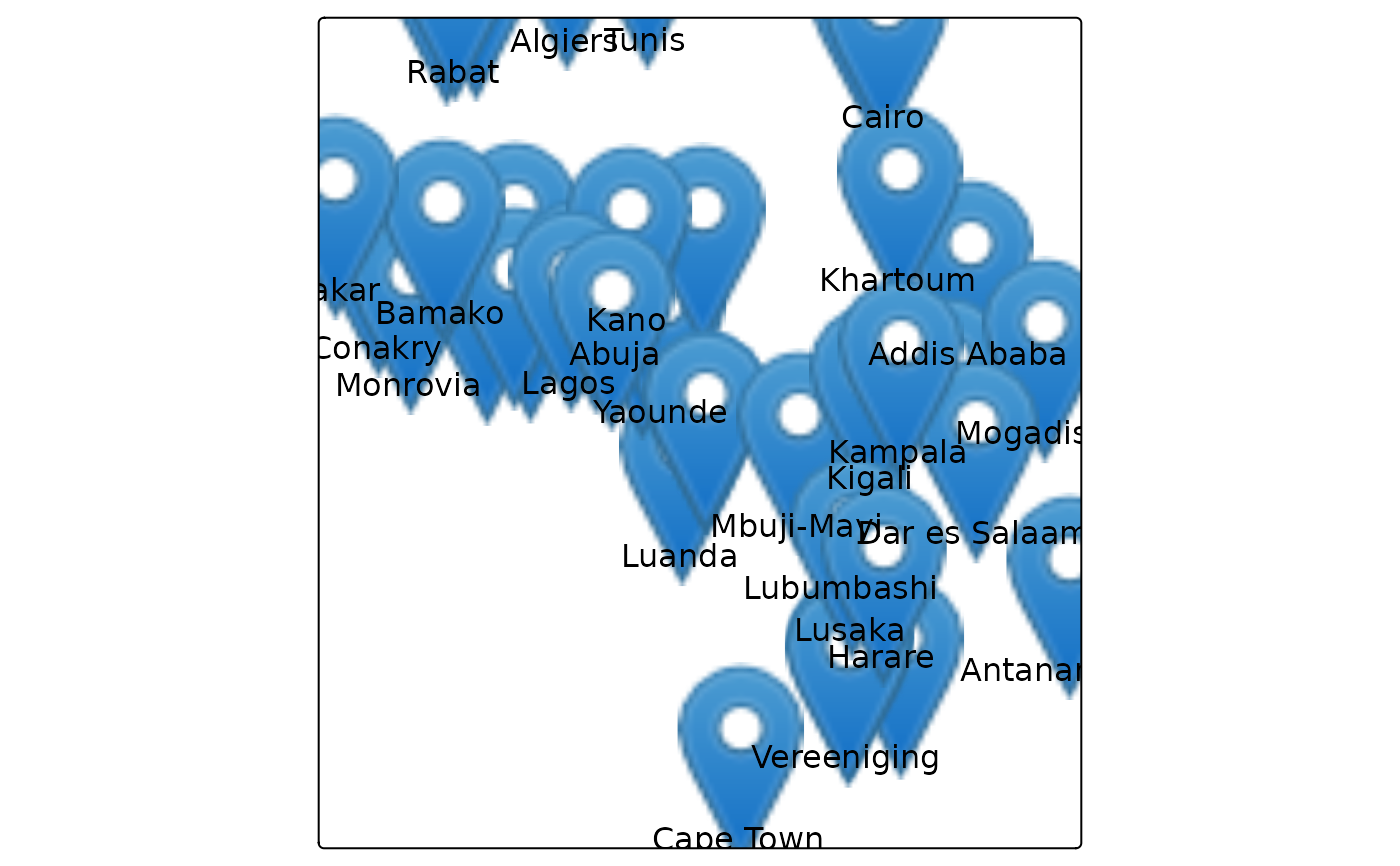Map layer that draws symbols Supported visual variables are: text (the text itself) col (color), size (font size), and fontface (font face).

## Usage

tm_text(
text = tm_const(),
text.scale = tm_scale(),
text.legend = tm_legend(),
text.free = NA,
size = tm_const(),
size.scale = tm_scale(),
size.legend = tm_legend(),
size.free = NA,
col = tm_const(),
col.scale = tm_scale(),
col.legend = tm_legend(),
col.free = NA,
col_alpha = tm_const(),
col_alpha.scale = tm_scale(),
col_alpha.legend = tm_legend(),
col_alpha.free = NA,
fontface = tm_const(),
fontface.scale = tm_scale(),
fontface.legend = tm_legend(),
fontface.free = NA,
fontfamily = "",
plot.order = tm_plot_order("AREA", reverse = FALSE, na.order = "bottom"),
trans.args = list(points.only = "ifany"),
mapping.args = list(clustering = FALSE),
zindex = NA,
group = NA,
group.control = "check",
...
)

## Arguments

text, text.scale, text.legend, text.free

Visual variable that determines the text. See details.

size, size.scale, size.legend, size.free

Visual variable that determines the font size. See details.

col, col.scale, col.legend, col.free

Visual variable that determines the col color. See details.

col_alpha, col_alpha.scale, col_alpha.legend, col_alpha.free

Visual variable that determines the border color alpha transparency. See Details.

fontface, fontface.scale, fontface.legend, fontface.free

Visual variable that determines the font face. See Details.

fontfamily

The font family. See gpar() for details.

Shadow behind the text. Logical or color.

plot.order

Specification in which order the spatial features are drawn. See tm_plot_order() for details.

trans.args, mapping.args

lists that are passed on to internal transformation and mapping functions respectively.

zindex

Map layers are drawn on top of each other. The zindex numbers (one for each map layer) determines the stacking order. By default the map layers are drawn in the order they are called.

group

Name of the group to which this layer belongs. This is only relevant in view mode, where layer groups can be switched (see group.control)

group.control

In view mode, the group control determines how layer groups can be switched on and off. Options: "radio" for radio buttons (meaning only one group can be shown), "check" for check boxes (so multiple groups can be shown), and "none" for no control (the group cannot be (de)selected).

...

to catch deprecated arguments from version < 4.0

## Details

The visual variable arguments (e.g. col) can be specified with either a data variable name (of the object specified in tm_shape()), or with a visual value (for col, a color is expected). Multiple values can be specified: in that case facets are created. These facets can be combined with other faceting data variables, specified with tm_facets().

The .scale arguments determine the used scale to map the data values to visual variable values. These can be specified with one of the available tm_scale_() functions. The default scale that is used is specified by the tmap option scales.var.

The .legend arguments determine the used legend, specified with tm_legend(). The default legend and its settings are determined by the tmap options legend..

The .free arguments determine whether scales are applied freely across facets, or shared. A logical value is required. They can also be specified with a vector of three logical values; these determine whether scales are applied freely per facet dimension. This is only useful when facets are applied (see tm_facets()). There are maximally three facet dimensions: rows, columns, and pages. This only applies for a facet grid (tm_facets_grid()). For instance, col.free = c(TRUE, FALSE, FALSE) means that for the visual variable col, each row of facets will has its own scale, and therefore its own legend. For facet wraps and stacks (tm_facets_wrap() and tm_facets_stack()) there is only one facet dimension, so the .free argument requires only one logical value.

## Examples

data(rivers)

tm_shape(rivers) +
tm_lines()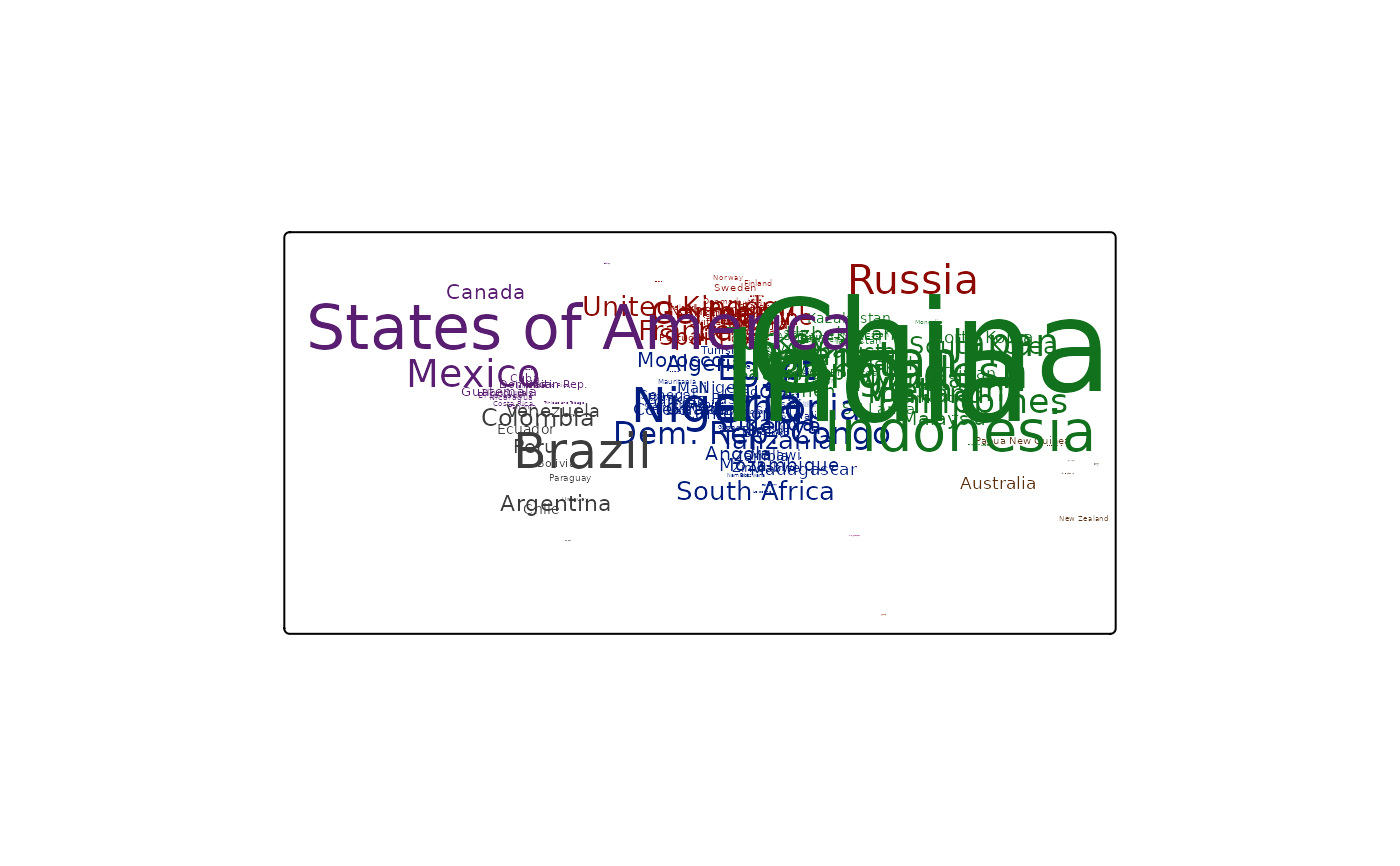tm_shape(rivers) +
tm_lines(lwd = "scalerank")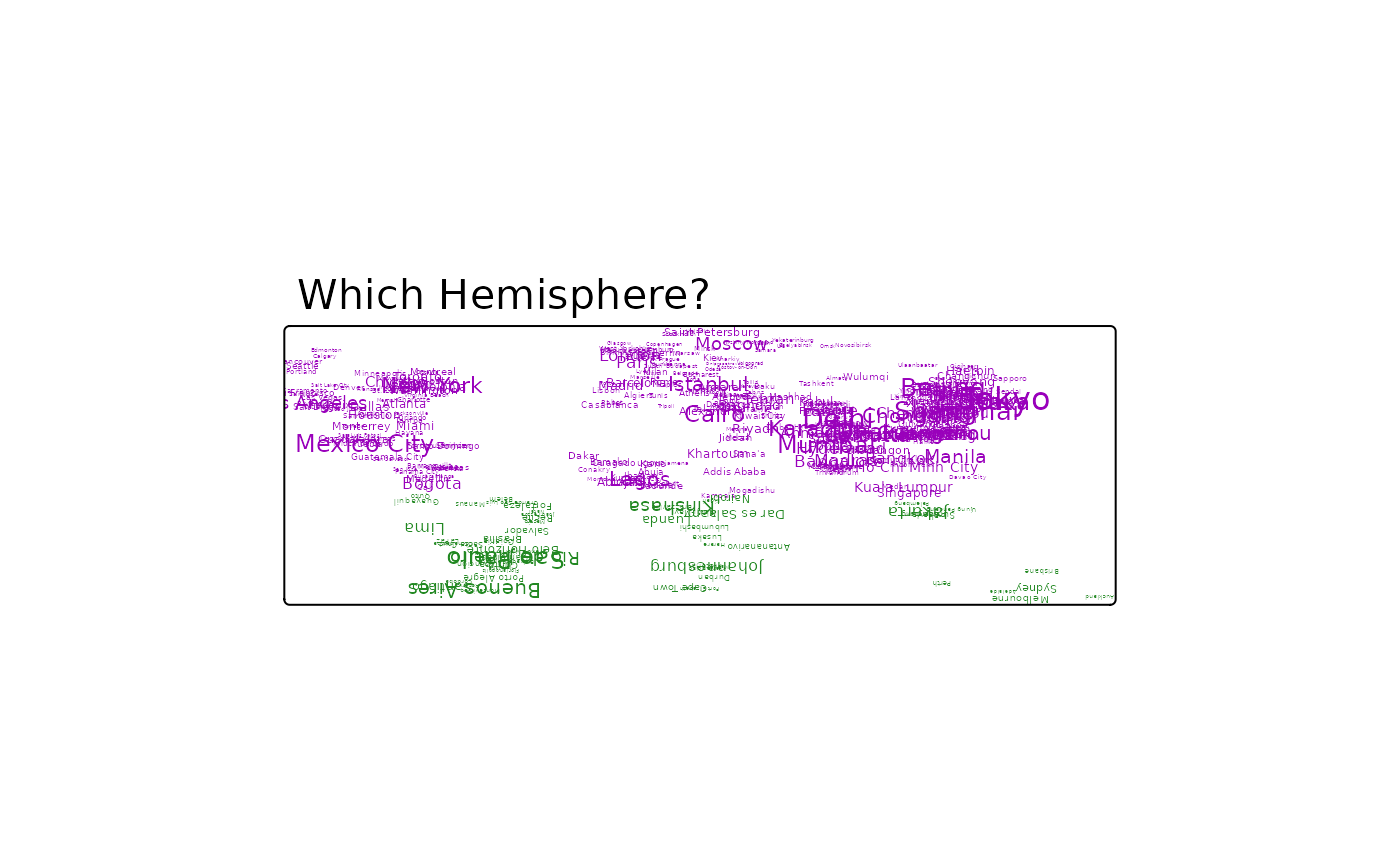tm_shape(rivers) +
tm_lines(lwd = "scalerank",
lwd.scale = tm_scale_continuous(values.scale = 2, n = 20),
lwd.legend = tm_legend(orientation = "landscape", item.width = 2),
col = "type",
col.scale = tm_scale(values = c("darkblue", "darkred")))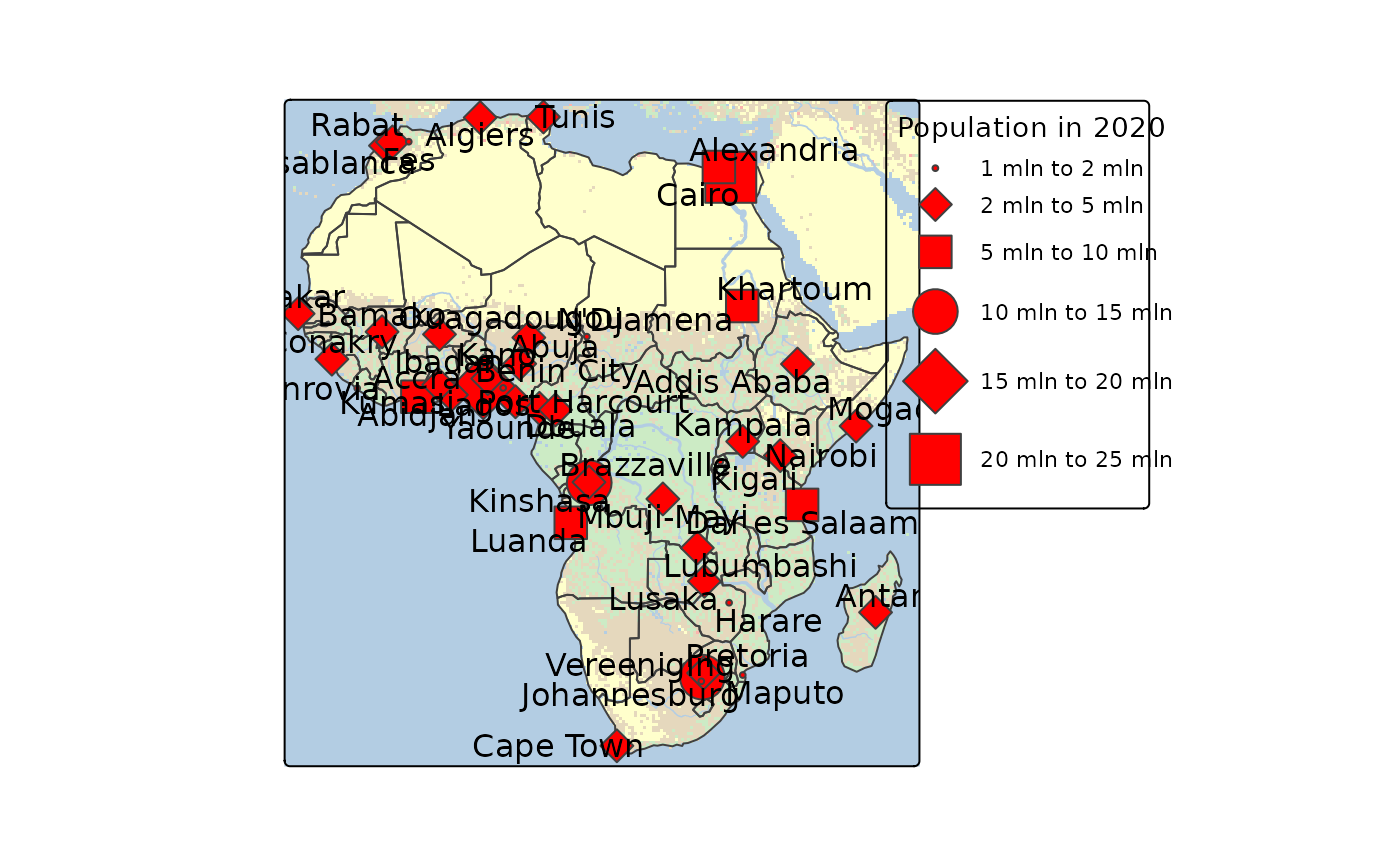tm_shape(rivers) +
tm_lines(lwd = "scalerank",
lty = "scalerank",
col = "scalerank",
col.scale = tm_scale_categorical(),
lty.legend = tm_legend_combine("lwd"),
col.legend = tm_legend_combine("lwd"))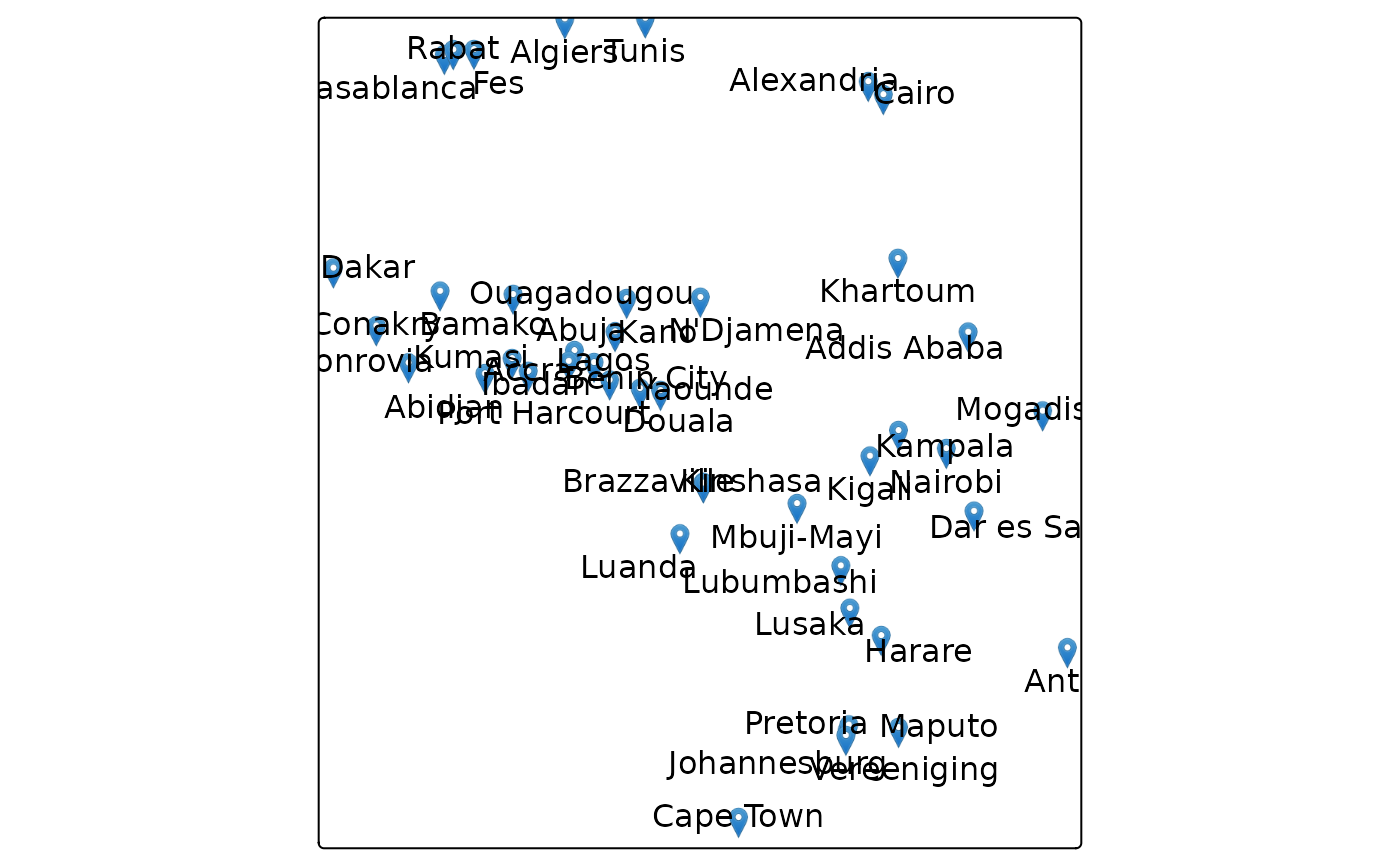### tmap3

tm_shape(World) +
tm_fill() +
tm_shape(rivers) +
tm_lines(col="black", lwd="scalerank", scale=2, legend.lwd.show = FALSE) +
tm_style("cobalt", title = "Rivers of the World") +
tm_format("World")
#> Deprecated tmap v3 code detected. Code translated to v4